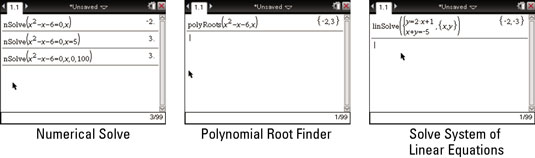##### TI-Nspire For Dummies, 2nd EditionPress [MENU]→Algebra to access the Algebra submenu from the TI-Nspire Calculator Application menu. The Numerical Solve command solves one-variable equations with ease. In the Catalog, the syntax for the Numerical Solve command is as follows:

`nSolve(Equation, Var[=Guess], lowBound, upBound)`

If you don’t specify the interval in which to look for a solution, TI-Nspire returns the value that’s closest to the origin. Using the Guess feature or specifying the interval helps you find the second solution of a quadratic equation. But wouldn’t it be nice to find both solutions to a quadratic equation using just one command?TI-Nspire CAS uses a Solve command that generates multiple solutions for given polynomial equations.

The Polynomial Root Finder command can find multiple roots to polynomial equations with just one command. To open the tool, press [MENU]→Algebra→Polynomial Tools→Find Roots of Polynomial. An easy-to-use wizard shows a dialog box to gather the necessary information and correctly enter the syntax into the Polynomial Root Finder command.

The Simultaneous Linear Equations Solver is another powerful tool in the Algebra submenu. To open the tool, press [MENU]→Algebra→Solve Systems of Linear Equations. Let the wizard guide you, and enter the system of equations that you want to solve.

Both the Polynomial Root Finder and the Simultaneous Linear Equations Solver let you manually enter the syntax or let the wizard guide you through the process.